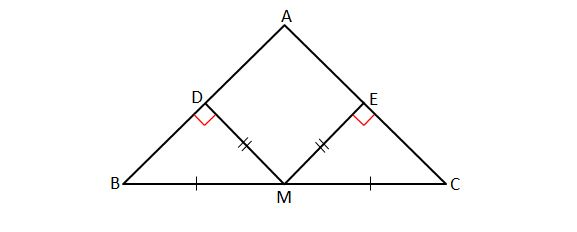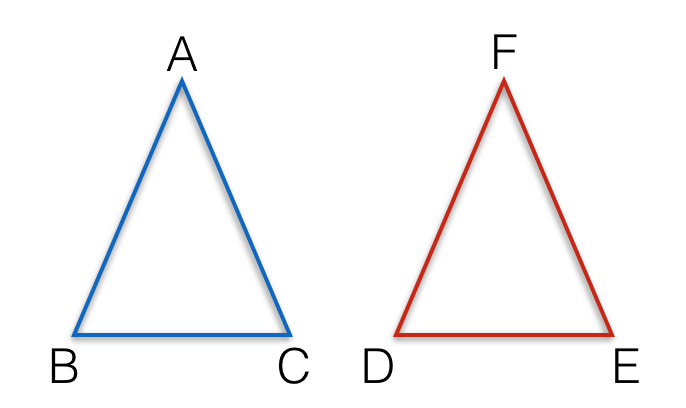Geometry

# Triangles - Identify Congruent TrianglesIn $\triangle ABC$ above, $\angle A={66}^\circ,$ $M$ is the midpoint of side $\overline{BC},$ and $D$ and $E$ are the feet of perpendicular drawn from $M$ to $\overline{AB}$ and $\overline{AC},$ respectively. If $\lvert{\overline{MD}}\rvert=\lvert{\overline{ME}}\rvert,$ what is the measure of $\angle CME ?$

Note: The above diagram is not drawn to scale.

Triangles $ABC$ and $DEF$ are congruent, that is, $\triangle ABC \cong \triangle DEF .$ If $AB=3$, $BC=4$ and $CA=5$, what is the length of $DE$?In triangles $ABC$ and $DEF$, if we know that $AB = EF, BC = DE$ and $\angle ABC = \angle DEF$, are the triangles congruent?

In triangles $ABC$ and $DEF$, if we know that $\angle ABC = \angle DFE$, $\angle BCA = \angle FED$ and $\angle CAB = \angle EDF$, are the triangles congruent?

Triangles $ABC$ and $DEF$ are congruent. If $AB = DE$, $BC = EF$, $\angle ABC = 37 ^ \circ$ and $\angle EDF = 39 ^ \circ$, what is the measure (in degrees) of $\angle EFD$?

×

Problem Loading...

Note Loading...

Set Loading...